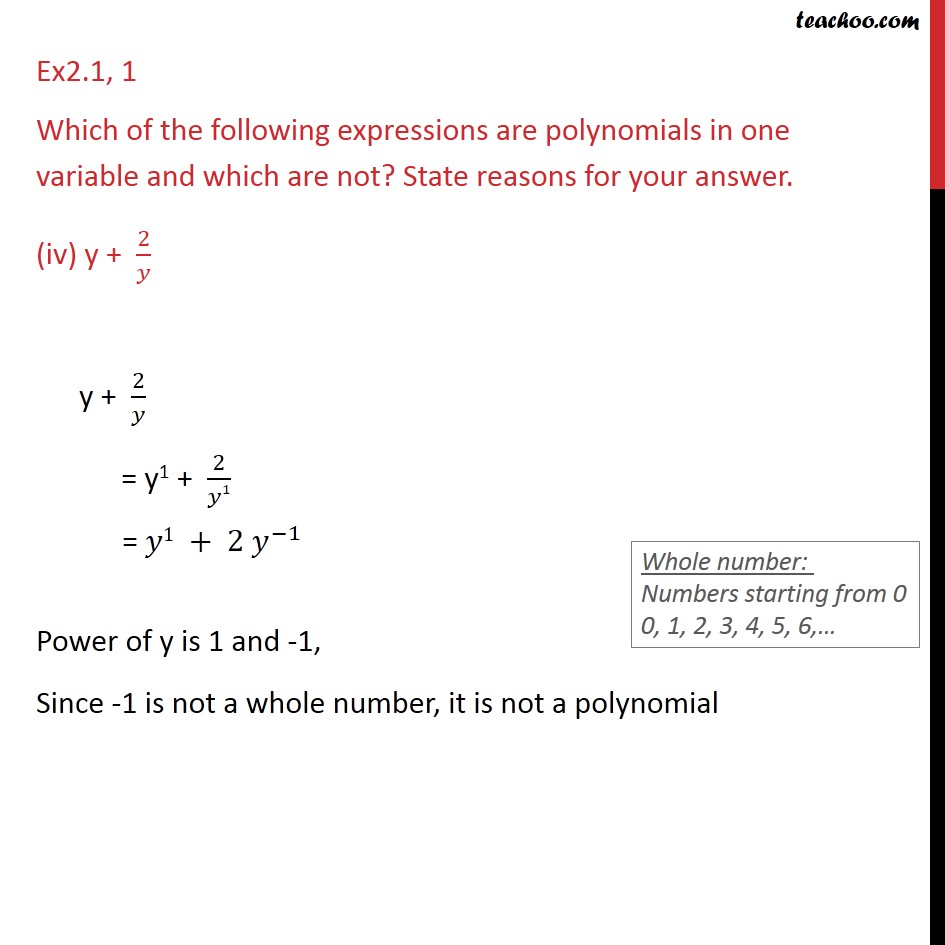1. Chapter 2 Class 9 Polynomials (Term 2)
2. Serial order wise
3. Ex 2.1

Transcript

Ex2.1, 1 Which of the following expressions are polynomials in one variable and which are not? State reasons for your answer. (iv) y + 2/𝑦 y + 2/𝑦 = y1 + 2/𝑦1 = 𝑦1 + 2 𝑦^(−1) Power of y is 1 and -1, Since -1 is not a whole number, it is not a polynomial